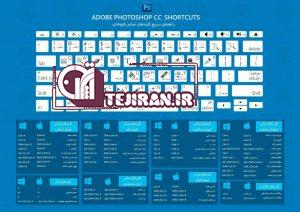# کلیدهای میانبر فتوشاپکلید میانبر در فتوشاپ:

ctrl+n = new
ctrl+o = open
ctrl+ w= close
ctrl+ s = save
ctrl+ z = undo
ctrl+c = copy
ctrl+x = cut
ctrl+ v = paste
ctrl+ f = paste in front
ctrl + b = paste in back
ctrl+ a = select all
ctrl+ d = transform again
ctrl+ ] = bring forward
ctrl+ [ = send backward
ctrl+ g = group
ctrl+ 2 = lock
ctrl+ 3 = hide selection
ctrl+ j = join
ctrl+ 7 = make
ctrl+ t = character
ctrl+ m = paragraph
ctrl+ e = apply last fillter
ctrl+ y = outline
ctrl+ h = hide edge
ctrl+ 0 = fit in window
ctrl+ 1 = actual size
ctrl+ r = show rulers
ctrl+ u = smart guides
ctrl+ ” = show gride
ctrl+ – = zoom out
ctrl+ + = zoom in
ctrl+ k = general
ctrl+ shift + s = save as
ctrl+ shift + p = print setup
ctrl+shift + z = redo
ctrl+shift + a = deselect
ctrl+shift + m = move
ctrl+shift + g = ungroup
ctrl+shift + t = tab rulers
ctrl+shift + o = creat outline
ctrl+shift + e = applay last effect
ctrl+shift + w = hide template
ctrl+shift + b = hide bunding box
ctrl+shift + ” = snap to gride
ctrl+alt + s = save as copy
ctrl+alt + p = document setup
ctrl+alt + 2 = unlock all
ctrl+alt + 3 = show all
ctrl+alt + j = average
ctrl+alt + b = make
ctrl+alt + 7 = relaese
ctrl+alt + 8 = release
ctrl+alt + e = last fillter
ctrl+alt + ” = sanp to point
shift f1 = help
shift f7= show align
shift f6= apperance
shift f9 = pathfinder
shift f5 = show style
shift f10 = trancparency
shift f8 = transform
f5 = show brush
f6 = show color
f7 = hide layers
f8 = show info
f9 = show gradiant
f10 = show stroke
f11 = show attributes

#توضیحات بیشتر برای میانبر ها

CTRL+O = Open file
CTRL+N = New file
CTRL+W = Close file (watch out for not mixing with CTRL+Q!!)
CTRL+S = Save file
CTRL+SHIFT+S = Save file As
CTRL+ALT+SHIFT+S = Save for web
CTRL+Q = Quit photoshop
CTRL+A = Select all
CTRL+D = Deselect
CTRL+E = Merge Down (more about layers later on..)
CTRL+R = Rulers visible (the things you drag guidelines out from..)
CTRL+T = Transform selection (Scale and rotate..)
CTRL+U = Change image Hue, Saturation and Lightness
CTRL+I = Inverse
CTRL+F = Repeat last used filter
CTRL+K = Preferences
CTRL+L = Levels
CTRL+Z = Undo (something you just can´t live without. Fact.)
CTRL+X = Cut
CTRL+C = Copy
CTRL+V = Paste
CTRL+B = Color balance
CTRL+M = Curves
CTRL+Space = Zoom In
CTRL+ALT+Space = Zoom Out
D = Set Colors to Default
X = Change Foreground color with Background color
CTRL+SHIFT+E = Flatten image
CTRL+SHIFT+T = Transform again
CTRL+SHIFT+U = Desaturate (make image black & white)
CTRL+SHIFT+I = Inverse selection (usefulnes=100000)
CTRL+SHIFT+P = Page setup
CTRL+SHIFT+D = Reselect
CTRL+SHIFT+F = Fade last filter..or thing you just did.. (works on alot of things)
CTRL+SHIFT+L = Auto levels
CTRL+SHIFT+Z = Redo
CTRL+SHIFT+N = New layerCTRL+ALT+0 = Actual pixels (“zooms” to 100%)
CTRL+ALT+O = As
CTRL+ALT+S = Save a Copy
CTRL+ALT+D = Feather selection
CTRL+ALT+F = Last filter, only you get to choose the stuff!!
CTRL+ALT+L = Auto levels, and… you get to choose.
CTRL+ALT+Z = History back (you´ve got to know this one!!!!)
CTRL+ALT+B = Auto-color-balance
CTRL+ALT+M = Auto-Curves
CTRL+SHIFT+ALT+Q = Quit photoshop..without asking anything!
CTRL+SHIFT+ALT+E = Flatten image to active layer